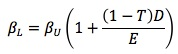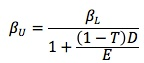### Seeing is believing!

Before you order, simply sign up for a free user account and in seconds you'll be experiencing the best in CFA exam preparation.

##### Subject 4. Estimating Beta

The determination of cost of capital under the CAPM approach involves the estimation of β, risk-free rate, and market return.

#### Estimating Beta for Public Companies

The historical β is the first step in the determination of the ex-ante β.

The standard procedure for estimating βs is to regress stock returns (Ri)against market returns (Rmi = a + b Rm, where b is the slope of the regression and corresponds to the β of the stock. It measures the riskiness of the stock.

To estimate βi an analyst needs to make several choices:

• Which index should be used to represent the market portfolio? The S&P 500 is a traditional choice to represent U.S. equities.
• What should be the length of data period and the frequency of observations?

According to Blume, there is a tendency of betas to converge towards the mean of all betas.

It corrects the estimated β for its tendency to revert to 1. It adjusts β in such a way that it is closer to the expected β in the future.

#### Estimating Beta for Thinly Traded and Nonpublic Companies

If we are thinking of a new company for a single project, we will have no historical records to go by. We would then compute the β of companies of the same size and about the same lines of business and after making necessary adjustments, take this as the β for the firm. The pure-play method can be used to take a comparable publicly traded company's beta and adjust it for financial leverage differences.

The β that we impute to a project is likely to undergo changes with changes in the capital structure of the company. If the company is entirely equity-based, its β is likely to be lower than it would be if it undertakes borrowing.

Let us call the β of a firm that is levered "levered β" and that of a firm on an all-equity structure "unlevered β."

β of a levered firm:where:
βL = β of a levered firm
βU = β of an unlevered firm
T = tax rate
D = component of debt in capital structure
E = component of equity in capital structure

If the β of a firm is available and that β has been estimated on the premise that the firm is unlevered, we can now ascertain the β of the firm should it undertake some borrowing by using the following formula:

β of an unlevered firm:In the same way, given the β of a firm which is already levered, we can ascertain what its β would be if it chooses an all-equity structure. This also means that if the target firm has leverage different from the structure assumed in estimating the levered β, this can first be converted into an unlevered β and then re-converted into a levered β using the leverage parameters relevant to the firm.

As a first step, we have to identify firms that reasonably resemble the project for which the beta is to be estimated. The stock β of these firms is then taken. Their respective leverage position (ratio of debt to equity) is also considered. After duly adjusting the tax factor and applying the above formula, we can determine the proxy β of the project assuming that it is unlevered.

The procedure is illustrated below:

Suppose there are three firms, P, Q, and R, which closely resemble project X (that is to be embarked upon). The stock betas of the three firms are taken and found to be 2.73, 2.23, and 1.73 respectively. The ratio of debt to equity for the three firms averages to 0.67. The marginal tax rate is 36%.

The average stock β works out to 2.23. Translating these numbers into the formula for unlevered firms, we get: βU = βL / (1 + (1 - T)(D/E)) = 2.23/(1+0.64 x 0.67) = 1.56.

This suggests that on an all-equity basis the β of the project would be 1.56. Now, if the project is proposed to be financed by 50% equity and 50% debt, we can modify the above β by applying the formula for levered firms:

βL = βU (1 + (1 - T) D/E) = 1.56 (1 + 0.64 x 0.5/0.5) = 2.56

So, on a 1:1 debt equity ratio, the β will be 2.56. This β can be used now for determining the cost of equity for the project and its weighted average cost of capital, to make a more meaningful appraisal.

Learning Outcome Statements

f. explain and demonstrate beta estimation for public companies, thinly traded public companies, and nonpublic companies;

CFA® 2022 Level I Curriculum, , Volume 4, Reading 30

User Comment
RamaG Highe debt, higher beta
leon121 unlevered beta = all equity beta; levered beta = beta with debt
leon121 i don't understand why these betas have to be labeled as is...when i've seen this referred to as Beta of Asset and Beta of Project
MathLoser Man this LOS is awesome. Especially the quiz
bushi Blume was the first one to write papers about the mean reversion of beta and came up with the adjusted beta. So 0.33 + 0.67 * beta was Blume's method.